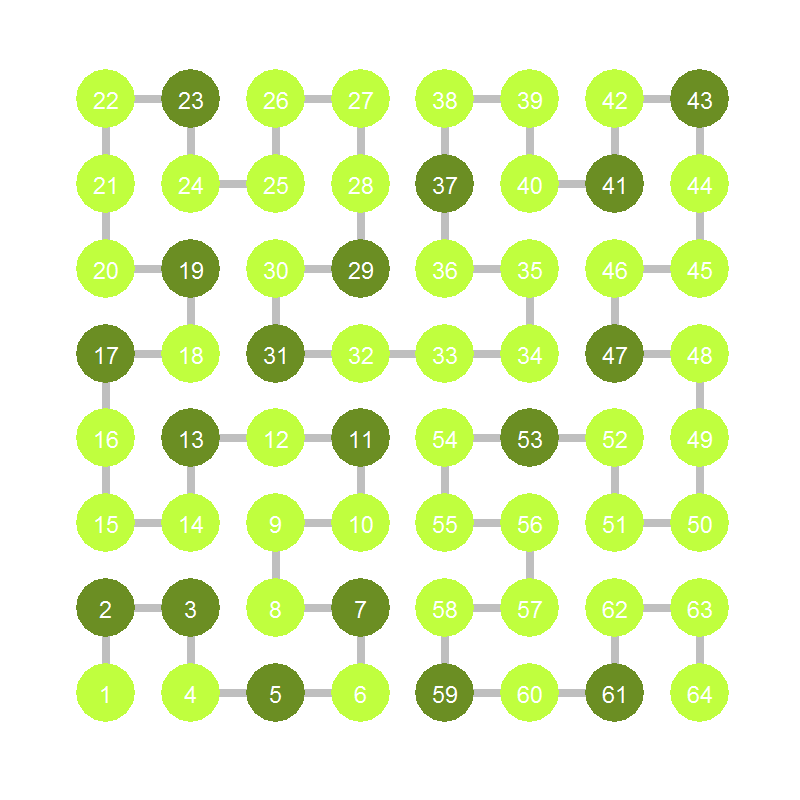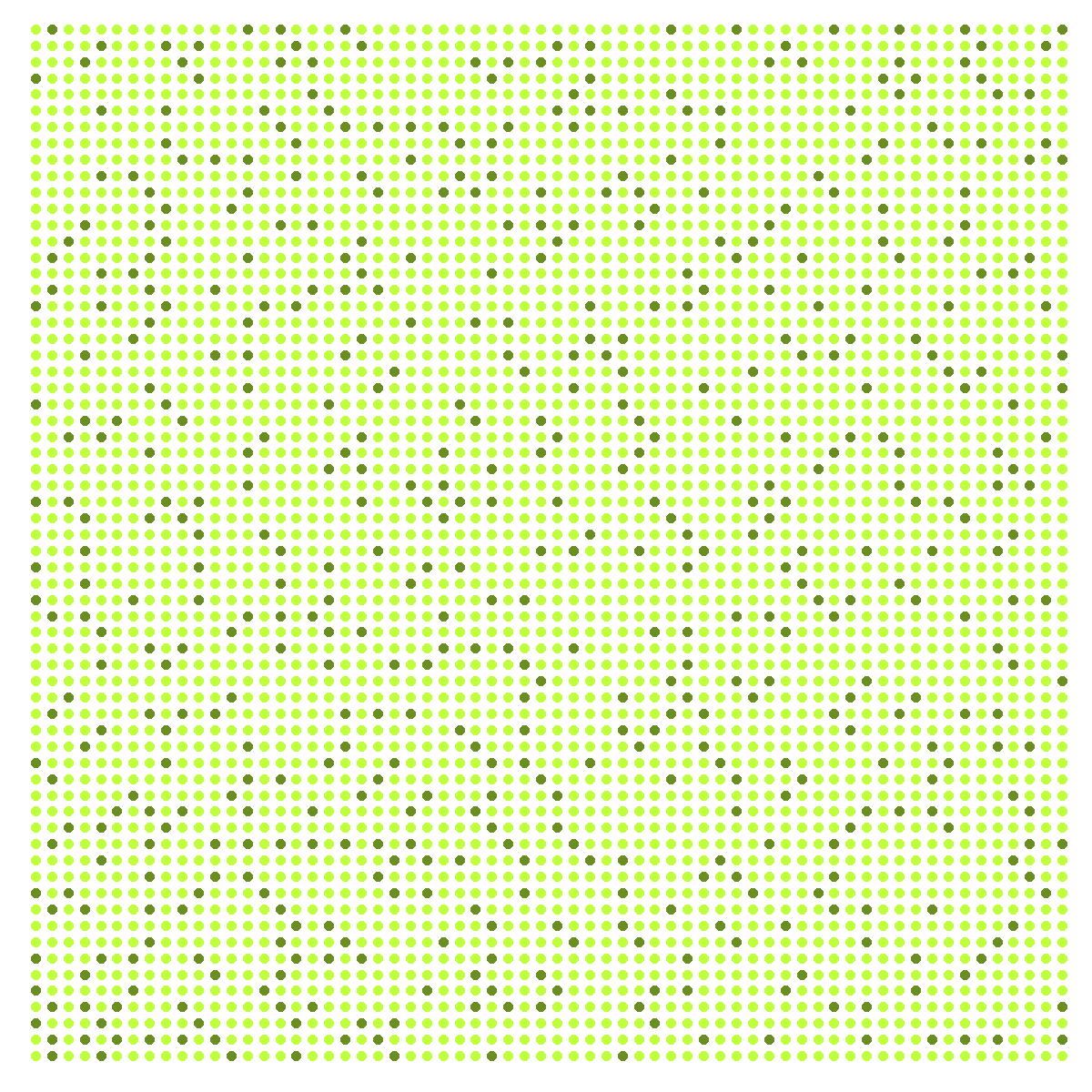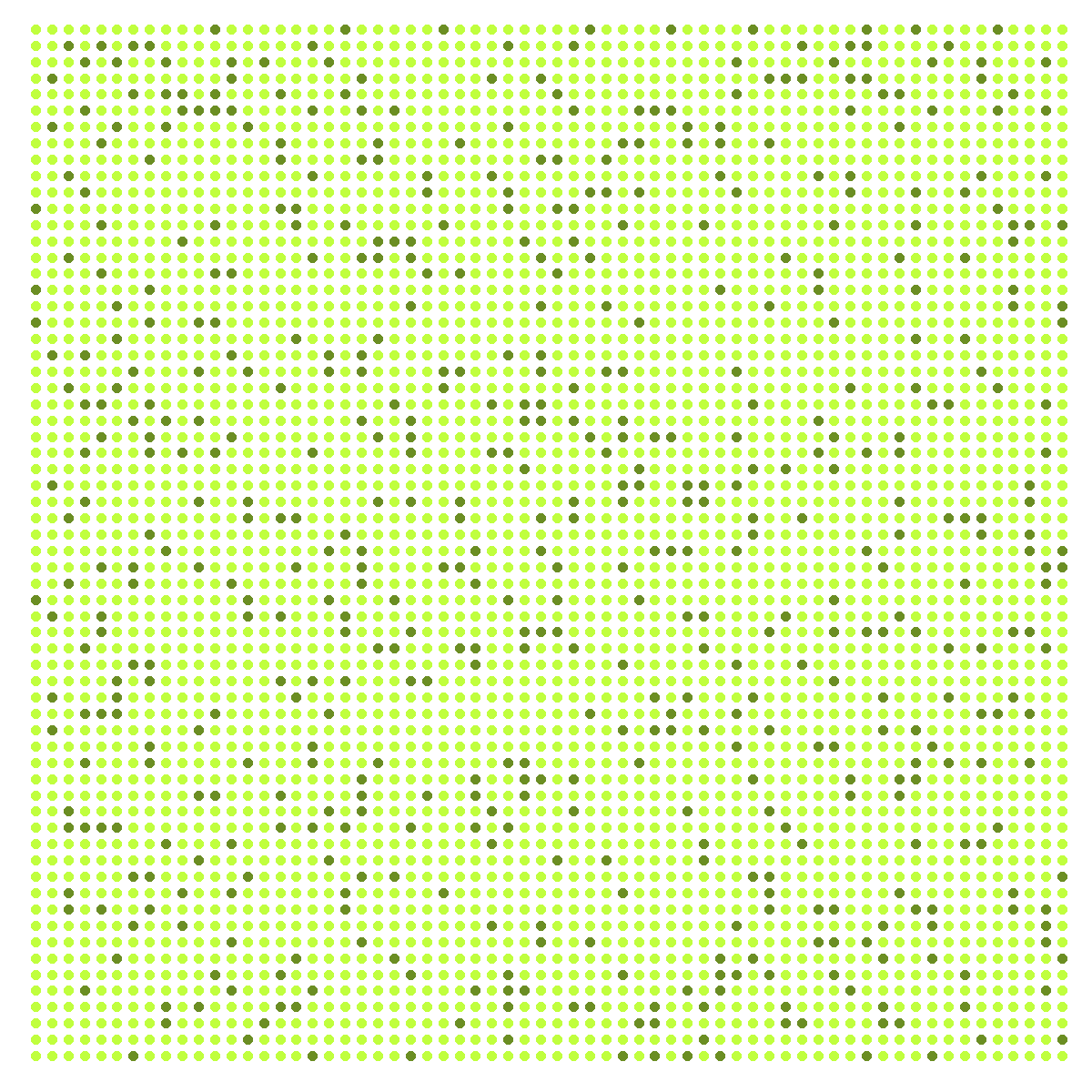# The Unbereable Insolence of Prime Numbers or (Playing to be Ulam)

So rock me mama like a wagon wheel, rock me mama anyway you feel (Wagon Wheel, Old Crow Medicine Show)

This is the third iteration of Hilbert curve. I placed points in its corners. Since the curve has beginning and ending, I labeled each vertex with the order it occupies:Dark green vertex are those labeled with prime numbers and light ones with non-prime. This is the sixth iteration colored as I described before (I removed lines and labels):Previous plot has 4.096 points. There are 564 primes lower than 4.096. What If I color 564 points randomly instead coloring primes? This is an example:Do you see any difference? I do. Let me place both images together (on the left, the one with primes colored):The dark points are much more ordered in the first plot. The second one is more noisy. This is my particular tribute to Stanislaw Ulam and its spiral: one of the most amazing fruits of boredom in the history of mathematics.

This is the code:

```library(reshape2)
library(dplyr)
library(ggplot2)
library(pracma)
opt=theme(legend.position="none",
panel.background = element_rect(fill="white"),
panel.grid=element_blank(),
axis.ticks=element_blank(),
axis.title=element_blank(),
axis.text=element_blank())
hilbert = function(m,n,r) {
for (i in 1:n)
{
tmp=cbind(t(m), m+nrow(m)^2)
m=rbind(tmp, (2*nrow(m))^r-tmp[nrow(m):1,]+1)
}
melt(m) %>% plyr::rename(c("Var1" = "x", "Var2" = "y", "value"="order")) %>% arrange(order)}
iter=3 #Number of iterations
df=hilbert(m=matrix(1), n=iter, r=2)
subprimes=primes(nrow(df))
df %>%  mutate(prime=order %in% subprimes,
random=sample(x=c(TRUE, FALSE), size=nrow(df), prob=c(length(subprimes),(nrow(df)-length(subprimes))), replace = TRUE)) -> df
#Labeled (primes colored)
ggplot(df, aes(x, y, colour=prime)) +
geom_path(color="gray75", size=3)+
geom_point(size=28)+
scale_colour_manual(values = c("olivedrab1", "olivedrab"))+
scale_x_continuous(expand=c(0,0), limits=c(0,2^iter+1))+
scale_y_continuous(expand=c(0,0), limits=c(0,2^iter+1))+
geom_text(aes(label=order), size=8, color="white")+
opt
#Non labeled (primes colored)
ggplot(df, aes(x, y, colour=prime)) +
geom_point(size=5)+
scale_colour_manual(values = c("olivedrab1", "olivedrab"))+
scale_x_continuous(expand=c(0,0), limits=c(0,2^iter+1))+
scale_y_continuous(expand=c(0,0), limits=c(0,2^iter+1))+
opt
#Non labeled (random colored)
ggplot(df, aes(x, y, colour=random)) +
geom_point(size=5)+
scale_colour_manual(values = c("olivedrab1", "olivedrab"))+
scale_x_continuous(expand=c(0,0), limits=c(0,2^iter+1))+
scale_y_continuous(expand=c(0,0), limits=c(0,2^iter+1))+
opt
```

## 2 thoughts on “The Unbereable Insolence of Prime Numbers or (Playing to be Ulam)”

1.spacedman says:

Excluding 2, all primes are odd. So on the Hilbert curve they’ll only appear on the odd-numbered points which form a regular grid. Hence you are more likely to see little diagonals and unlikely to see solid lines, which you will see on the random colouring. Try again with only odd numbers!

1.Brian Stamper says:

I tried it, and I have to agree. The random set looks more “ordered” if you only choose odd numbered points. Here i just double the weight of ‘TRUE’ and then set all the odds to false, to get about the same number of points:

mutate(prime=order %in% subprimes, random=sample(x=c(TRUE, FALSE), size=nrow(df), prob=c(2*length(subprimes),(nrow(df)-length(subprimes))), replace = TRUE))
df\$random[(1:(nrow(df)/2))*2] <- FALSE